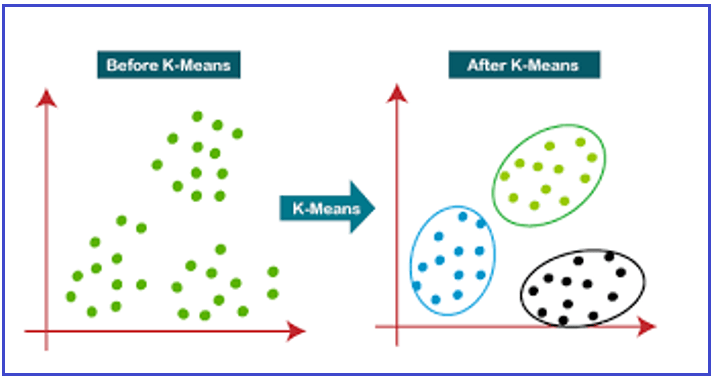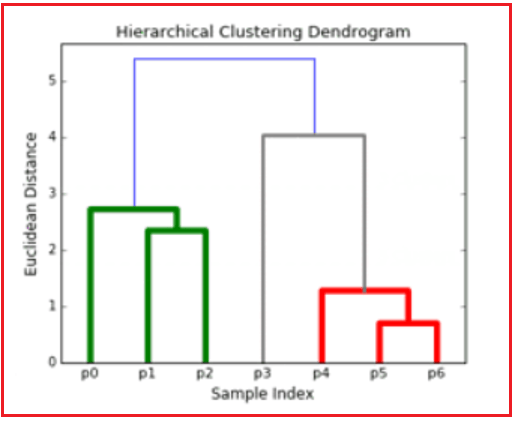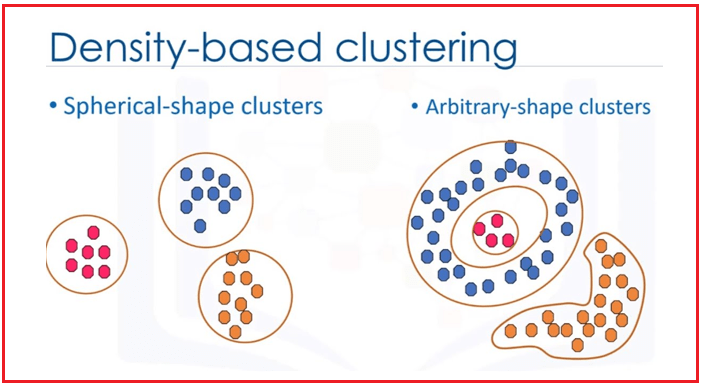# Clustering in Machine Learning

## Clustering in Machine Learning

In this article, I am going to discuss Clustering in Machine Learning with Examples. Please read our previous article where we discussed Random Forests in Machine Learning with Examples.

##### Clustering in Machine Learning

Clustering is a Machine Learning approach that groups data points together. We can use a clustering algorithm to classify each data point into a specific group given a set of data points. In theory, data points belonging to the same group should have comparable attributes and/or features, whereas data points belonging to other groups should have very distinct properties and/or features.

Clustering is an unsupervised learning strategy that is widely utilized in various domains for statistical data analysis.

When applying a clustering algorithm to our data, we can utilize clustering analysis to acquire some key insights from our data by seeing what categories the data points fit into. Here are major clustering algorithms –

1. K-Means Clustering
2. Hierarchical Clustering
3. Density-Based Spatial Clustering of Applications with Noise (DBSCAN)

Clustering has a huge number of applications in a variety of fields. Clustering is used in a variety of ways, including:

• Engines that make recommendations
• Segmentation of the market
• Analyzing social networks
• Grouping of search results
• Imaging in medicine
• Segmentation of images
• Detecting anomalies

Let’s now have a look at the above-mentioned Clustering algorithms in detail –

##### K-Means Clustering in Machine Learning-

The most well-known clustering algorithm is K-Means. Many beginnings data science and machine learning classes cover it. The K-means algorithm is an iterative clustering algorithm that seeks out local maxima in each iteration.

1. To begin, we’ll choose a few classes/groups to use and then randomly initialize their center points. Take a cursory glance at the data and try to spot any significant groupings to determine the number of classes to utilize. The center points, which are the “X’s” in the figure – Iteration 1, are vectors of the same length as each data point vector.
2. Each data point is categorized by calculating the distance between it and the center of each group and then placing it in the group with the center closest to it.
3. We recompute the group center based on these classed points by taking the mean of all the vectors in the group.
4. Repeat these processes for a specified number of iterations or until the group centers do not change significantly between iterations. You can also choose to initialize the group centers at random a few times and then pick the run that appears to have produced the best results.##### Hierarchical Clustering in Machine Learning

Hierarchical Clustering is a clustering technique that splits a data set into a number of clusters without requiring the user to indicate the number of clusters to be formed before the model is trained. Connectivity-based approaches are another name for this type of clustering technique. Simple partitioning of the data set is not performed in this method, but it does present us with a hierarchy of clusters that merge after a certain distance.

After the dataset has been hierarchically clustered, the outcome is a tree-based representation of data points [Dendogram] that is separated into clusters.##### Density Based Clustering in Machine Learning

Technique clusters will be generated by segregating distinct density zones depending on varied densities in the data plot in this clustering. The most commonly used algorithm in this sort of method is DBSCAN (Density-Based Spatial Clustering and Application with Noise). The primary principle behind this technique is that each point in the cluster should have a minimum number of points in the neighborhood of a particular radius. If you look closely at the above-mentioned clustering algorithms, you’ll see that they all have one thing in common: the shape of the clusters generated is either spherical, oval, or concave.

DBSCAN can create clusters of various shapes; this algorithm is best suited to datasets with noise or outliers. After training, this is how a density-based spatial clustering method looks.In the next article, I am going to discuss Similarity Metrics in Machine Learning with Examples. Here, in this article, I try to explain Clustering in Machine Learning with Examples. I hope you enjoy this Clustering in Machine Learning with Examples article.